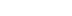/
article

# Water interaction model for boats in video games

Avalanche Studios senior software engineer Jacques Kerner (Just Cause 3) walks us through coding "a simplified model that captures the important features of a boat" in this meaty programming article.

Jacques Kerner is a senior software engineer at Avalanche Studios.

## Let's talk vehicle physics!

We don't talk enough about vehicle physics for video games. Articles on the net about vehicle physics for video games are few and far between, and are usually about how to get started. A video game vehicle programmer today finds herself or himself in a relative vacuum. Maybe it is because it seems too complicated to explain, or we are ashamed to expose the hacks, simplifications and shortcuts we make compared to 'proper' realistic simulations. Whathever the reasons are, video games have unique constraints when it comes to simulating vehicles, and this makes it worth writing about. It is a fascinating subject that mixes physics, camera work, audio, special effects, but also human perception and even psychology.

I chose to start talking about boats because, well, I recently worked on them, but also because I found that their dynamics is not fully understood even at the research level (although a lot is understood). When it is, the models or theories are formulated in such a way that makes them hard to apply directly to video games. Or they require very expensive simulation methods that are practically impossible to control and adapt to the capricious needs of designers and gamers. But it is possible to write a simplified model that captures the important features of a boat. There is definitely an art to it, a scary leap of faith involved and quite a bit of creative physics that would have a Kelvin or a Stokes roll in their graves.

## Series on boat physics

In this series, I present an algorithm for calculating the most important forces acting on a boat in water. The main motivation is to develop a model which captures the major dynamic traits of boats in water, yet avoids resorting to complex and expensive fluid dynamics computation.

I constrain myself to a reasonable performance budget, say less than 1 ms per boat. The model must be robust enough to simulate boats of a wide variety of sizes and shapes evolving in calm to very stormy waters.

The first article in this series will be dealing with hydrostatic forces, but will lay an important foundation for calculating all the other forces involved in this model. The other forces are dynamic forces caused by the motion of the boat relative to the water. They will be the subject of articles to follow.

## Buoyancy Force 101

Before I dive in the algorithm itself, I want to review a bit about buoyancy. All we need to be able to do is to calculate the magnitude and point of application of the buoyancy force on an partially submerged body.

When a body is submerged in a fluid, the fluid exerts a force on the surface of the body, due to the pressure in the fluid. The bigger the pressure, the bigger the force. The force is the result of the many water particles in movement in the fluid, colliding against the surface of the body in an elastic way - i.e. like perfect billiard balls. It is a microscopic force, its effect is felt even if the water isn't flowing in any particular direction (current) or if the boat stays still, and it is therefore called the hydrostatic force. The net force of all these atoms or molecules hitting the surface is perpendicular to the surface. One other thing to note is that the pressure in water increases with depth (on a planet with gravity anyway), because a greater depth implies that more and more water is pressing down with its weight. However pressure doesn't have a preferred direction in itself, and even if there is no fluid directly above a point in water, the pressure at the point will still be dependent on the overall depth nearby. (*)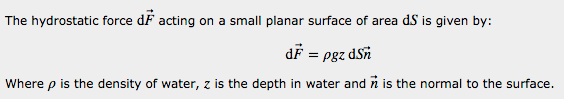The increasing force with depth is very important for buoyancy, because the overall buoyancy force is due to an imbalance in the vertical component of the hydrostatic forces on the surface of a body. The horizontal component of the hydrostatic forces all cancel out. Intuitively, this is because for every given small surface of the (closed) volume, you can always find another small surface facing exactly the opposite direction at the same depth. Since the magnitudes of the hydrostatic forces are the same but applied in opposite directions, they cancel out. On the other hand, the vertical component of the hydrostatic forces do not cancel out at all. Overall, because the volume is closed, surfaces which normal is generally pointing down will be found at greater depths than surfaces which normal is generally pointing up. So the pressure forces on surfaces which normal is pointing down prevail. The pressure force being in the opposite direction to the normal, the resulting force is pointing up and it can be shown  that its magnitude is equal to "the weight of the displaced fluid", meaning the weigth of the volume of the body if it were filled with water.

Now we are still missing one piece of the puzzle: to have everything we need to work on buoyancy, we also need the point of application of the hydrostatic force. The point of application of the buoyancy force is the point with respect to which the moments of all hydrostatic forces cancel out. If we continue reasoning in terms of small elementary surfaces on the submerged body, things become a little less obvious. Since the hydrostatic force gets larger the deeper you go, the point of application of the hydrostatic force on a given non horizontal surface is generally found lower than the center of the surface. As shown in Appendix A, on a submerged triangle, which is particularly useful in games since we tend to triangulate everything, the point of application is always lower than the center. Yet somehow the sum of all the moments of all these forces applied generally lower than the centre of any surface still cancel out around the centroid of the volume. A formal proof of this is found in  by applying the Ostrogradsky-Gauss theorem (**), or divergence theorem. It can also be verified numerically. The reason I mention this is that if you were to divide the body in small surfaces, say triangles, and sum all the hydrostatic forces and their moments, you could be tempted to make the simplification to calculate the moments as if the elementary forces on each triange were applied at its center (easy to determine). If you do so however, you will not get the correct result. You will get the correct force, but you will get a residual moment around the center of the volume submerged, and the boat may tip on one side at rest, even on perfectly flat water. This is especially true if you use a low polygon count mesh to reduce performance cost, as the error made on each triangle is then relatively important. On the other hand, if you have many small triangles, the error caused by the simplification will be reduced drastically and the simplification may become acceptable. But there is a trade-off between the complexity of the computation of the correct center and the number of triangles in the hull. Appendix A gives the formula for the location of the point of application of hydrostatic forces on a submerged triangle.

## Two ways to flip a boat

In light of what we just reviewed, there are two ways buoyancy forces can be calculated. The volumetric method: by evaluating the volume submerged and determining its centroid. The surfacic method: by determining the surface submerged, and calculating the force applied on it. The two methods, if applied correctly, should give the same result.

Both the volumetric and surfacic methods, without too much approximation, require us to determine the intersection of the water with the hull. This can be intimidating, especially when considering non flat water surface: it sounds expensive and complicated. This may be why the use of simple volume primitives is tempting to many. For instance, spheres: in contrast to intersecting a complex shape with the surface of the water, calculating the volume of a portion of a sphere submerged in water is fast and easy if the water can be approximated as planar around it. It can even be determined analytically, which means that, in theory at least, it is of infinite precision, or as precise as floating point operations will allow (yes, so many puns in this article). It also looks like the volume submerged will change in a continuous, progressive manner as the body moves in and out of the water, and continuity of a physical model is often desirable in games. But approximating the hull of a typical boat with spheres can quickly turn into a nightmare, as many spheres of different sizes may be needed. Because spheres are one of the worst choices for densely packing a volume, you will be left with significant gaps in between the spheres (figure 1). There is an upper bound to how densely the volume can be packed, even with spheres of different radii . The presence of these gaps leads to noticeable irregularities in buoyancy. Spheres can also be made to overlap, but then the volume submerged will be overestimated. Finally, while it is easy to compute the intersection of a planar surface with a sphere, calculating the intersection of a sphere with an arbitrary water surface is much more work, and we might as well try and find a solution to intersecting the hull with water.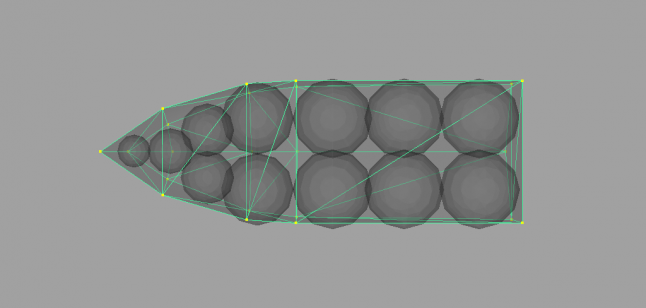Figure 1 - Approximating the volume of a boat with spheres is not the way to go.

The volume of the body could also be voxelized, i.e. approximated by a collection of simple volume primitives like cubes. The voxels which happen to be intersected by water could be further voxelized, till a certain precision is reached. The problem with volume approximations is that they give a (somewhat coarse) answer to the question "what is the amount of water displaced?", but are pretty much useless in terms of determining the waterline of the object immersed, which is useful for instance for water special effects such as splashes and foam, or for determining the shape of the surface in contact with water, unless you use an obscene amount of them.

Assuming we could compute accurately the surface of the hull submerged in water, we still have the choice between the volumetric and the surfacic approaches. With the volumetric approach, we must close the submerged surface of the hull to form a closed volume, compute its total volume and centroid, and apply the buoyancy force there. With the surfacic approach, we would calculate the hydrostatic pressure forces at each submerged surface element (triangles), and sum their linear and angular impulses around the center of gravity of the body.

The advantage of the surfacic method is that it is not necessary to close the volume, everything is prepared to directly sum forces. With the volumetric approach, the volume submerged could also consist of more than one volume. It's easy to see in the case of catamaran hulls for instance. But even if the boat doesn't have holes, the intersection with water could represent two or more volumes to close, and we would have to determine which submerged triangles contribute to which volume, which represents an additional complication to that approach. The surfacic approach is more robust in that respect, as it works regardless of the number and shape of volumes formed and doesn't require any closing. It is the one I chose for the algorithm.

## Structure of the algorithm

I'm now going to outline the structure of the algorithm with some of the key simplifications that allow it to run fast, yet give adequate results.

The first assumption I make is that the surface of the water is described by a triangle mesh of some sort, which vertices move each frame with the motion of the water. It is not always the case of course, but it is always possible to approximate the surface of the water by a triangulated mesh. Later in the article, I describe how to sample the water surface and prepare a triangulated mesh representation for it around the body.

The main objective is to determine the intersection between the surface of the water and the surface of the hull. After seeing the implementation by Edouard Halbert , I started by implementing an accurate solution taking into account all cases of the water surface intersecting a triangle. This problem is somewhat complicated because in theory there are lots of ways that a surface can segment a triangle. The surface could cut one triangle in several places, go through the center without intersecting any edges, or submerging any vertices. Each such submerged region needs to be triangulated, but those regions are not necessarily convex, so are harder (and slower) to triangulate. Furthermore, these cases are relatively common. Even in relatively calm waters they are very quickly encountered, and need to be properly handled in a way that doesn't cause unrealistic discontinuities in the amount of surface considered submerged. After some effort spent implementing a perfectly accurate but very slow intersecting algorithm it became clear that I needed to find ways to simplify the algorithm without sacrificing too much of the general behavior. The algorithm I present here is the result of these simplifications. I will not present details of the first accurate algorithm because it is extremely tedious and boring, and ultimately the optimized algorithm works just fine and runs an order of magnitude faster.

The structure of the optimized algorithm is as follows: the floating body is approximated by a triangulated mesh: the hull. We determine the height above water of each vertex of that hull. If the height above water is negative, the vertex is submerged. Triangles which three vertices are above water are considered to be entirely out of the water. This is a simplification, in reality the water could be above some part of the triangle but below all three vertices, as show in figure 4. Likewise, we consider a triangle to be fully submerged if all three vertices are under the water, even though some part of the water could be sinking under the triangle in some of its area. When only one or two vertices are under water, we cut the triangle into one region under the water and one region over, as shown in figure 2. If the region under the water is not a triangle, we further triangulate it. I am making the bold (and theoretically incorrect) assumption that the surface of the water is cutting the edge only once between a submerged vertex and a vertex out of the water. Figure 3 shows some examples of cases not accurately intersected. We end up with a list of triangles, all of which are under water. We then calculate the hydrostatic and hydrodynamic forces acting on these triangles.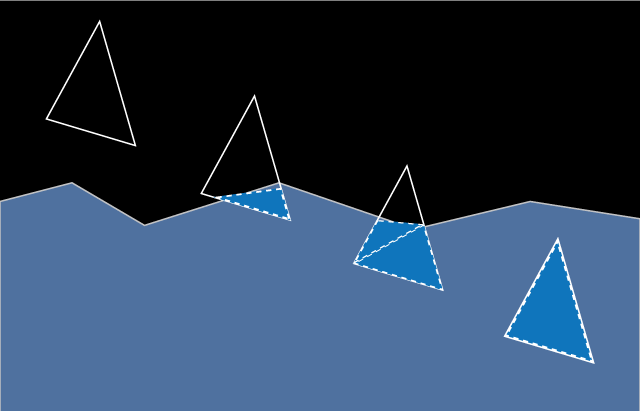Figure 2 The 4 simplified cases of triangle intersections with the water patch. From left to right, triangle with respectively 0, 1, 2 and 3 vertices submerged. With 2 vertices submerged we need to further triangulate the part submerged. Notice that the intersection with the water is not exactly accurate. It is due to another simplification which we will explain.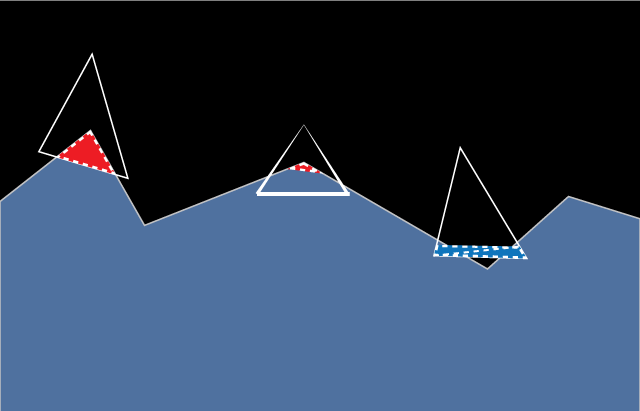Figure 3Three examples of cases mishandled by the optimized algorithm. The red areas denote triangles which should have been considered under water, yet were missed. The two triangles on the left have intersections with the water, but none of their vertices are under water. The triangle in the middle is seen in perspective, it doesn't even intersect the surface of the water on any of its edges, as the crest of the wave punches through the middle of the triangle. The triangle on the right has two vertices below the water, but the water also leaves the triangle at the edge between these two vertices.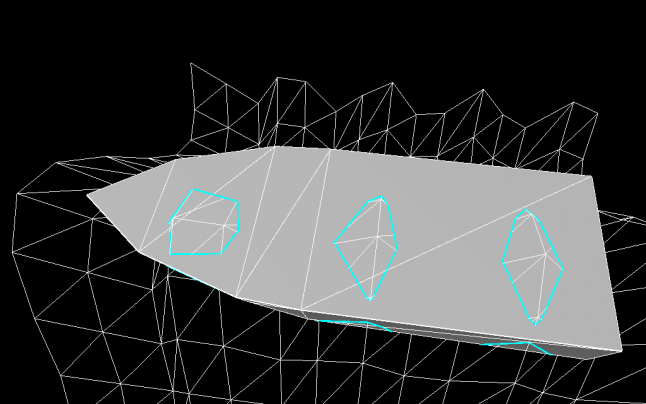Figure 4 - An example of a case happening often with choppy water. The bigger lower right triangle of the hull is intersected in several regions, one of which does not intersect any edges. Worse than that, the intersected regions could have been concave, making them harder to triangulate quickly. Supporting all such cases consumes both development time and performance at runtime. Ultimately, it is somewhat pointless since, in all rigor, the surface of the water is itself modified by the presence of a boat.

For a boat model, those cases are less important than it seems. The sacrifice in accuracy is not a problem in practice, as long as the size of triangles of the hull is not too big compared to the amplitude and wavelength of the smallest waves we are interested in.

The main advantage of the proposed approach is that all vertices can be processed in a first pass, regardless of which three form a triangle. All the information is then available to process each triangle, which gives rise to 0, 1 or 2 submerged triangles. The triangle intersection part is very simple and fast. Most of the processing lends itself nicely to parallelization if need be. We also know the maximum number of submerged triangles we can get: twice the number of triangles in the body hull. This allows us to pre-allocate all the memory beforehand in a simple array.

In the next section, we present important details of implementation, such as how to approximate the water surface to optimize the water depth query, how exactly to cut a triangle which has only one or two vertices under water and how the buoyancy forces are calculated.

## Details of implementation

### Water patch

To determine the height above water of each vertex, we need a fast way to determine the position of the water under a given point. Here a lot depends on the way water is simulated in the target game or simulation. If the water is flat or described by a simple function, it may be fast to simply determine the height of the water by directly sampling it or evaluating it, at every query. In other cases though, the algorithm to determine the height of the water is expensive and only a limited number of queries can be made. It is the case of Fast Fourier based methods for instance, such as Tessendorf waves . In such cases, I suggest sampling the water once and for all at equally spaced points around the body, therefore creating a height map which is used for all subsequent height queries. I'll refer to this height map as thewater patch. The water patch needs to be at least as big as the vertical projection of the body. For instance, you can start with a square water patch which side is as long as the diagonal of the bounding box of the body. As in a traditional height map, the water patch consists of a rectangular area subdivided in bands which form rows and columns, intersecting at square cells (figure 5 and 6). Each cell is itself separated in 2 triangles. For each triangle, we compute the equation of the plane supporting it, which makes it very fast to determine the projection of a point onto it.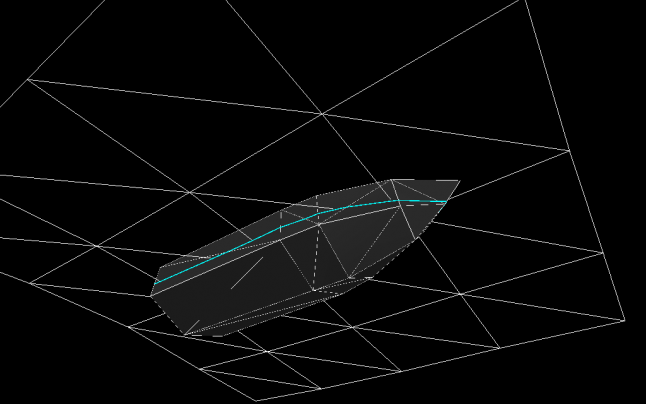Figure 5 - A 4 by 4 water patch and the water line (cyan) seen from below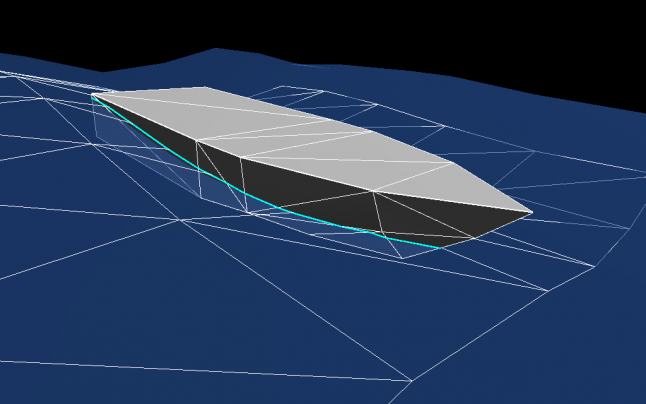Figure 6 - A 5 by 5 water patch and the water line (cyan) seen from above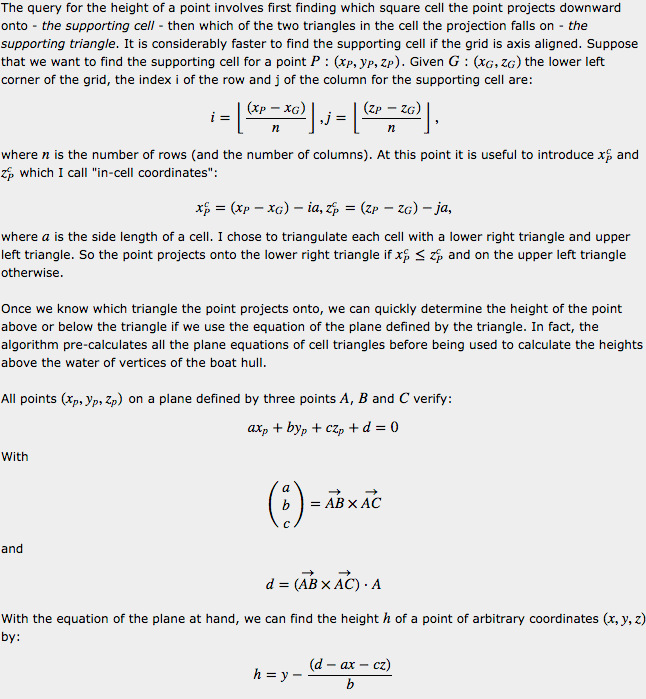### Cutting algorithm

When a triangle has some of its vertices under the water and some over, we need to cut the triangle into a portion which is fully over the water, and a portion fully under the water. There is a way to simplify the cut which is fast to calculate and continuous in behavior. By "continuous in behavior", I mean that we want to avoid situations where a small change in vertices height introduces sudden large changes in the submerged area. This problem wouldn't happen if we were accurately cutting the triangles in parts under and parts over the water, it is solely due to the approximation, and we need to find one which behaves nicely. This problem was one of my earlier setbacks, and would sometimes introduce instability in buoyancy where the body would suddenly jump or sink in noticeable ways, ruining the overall effect.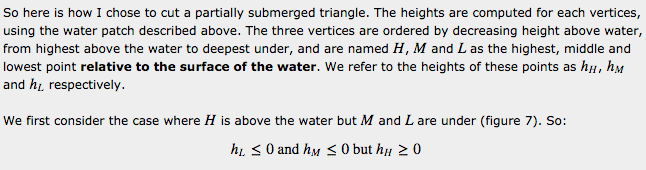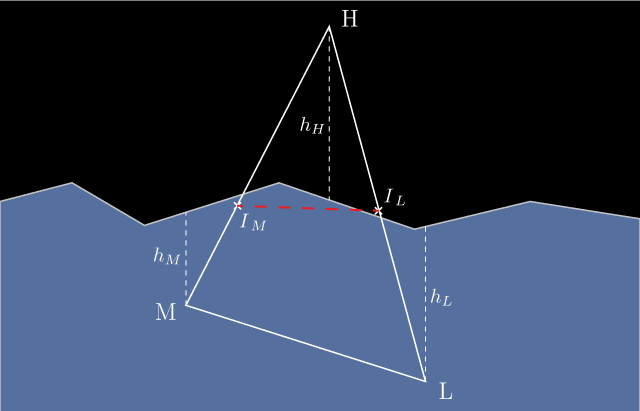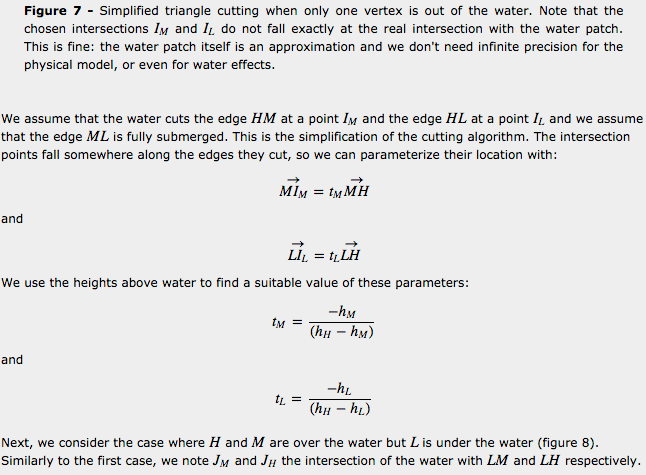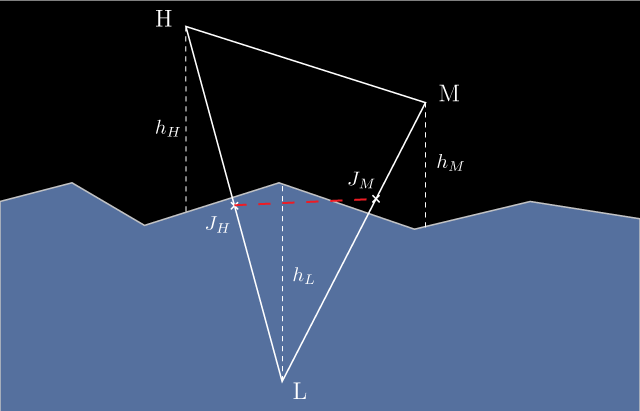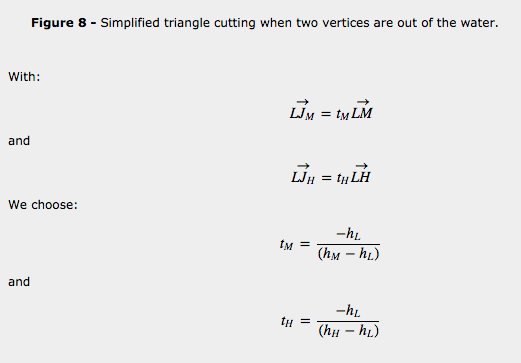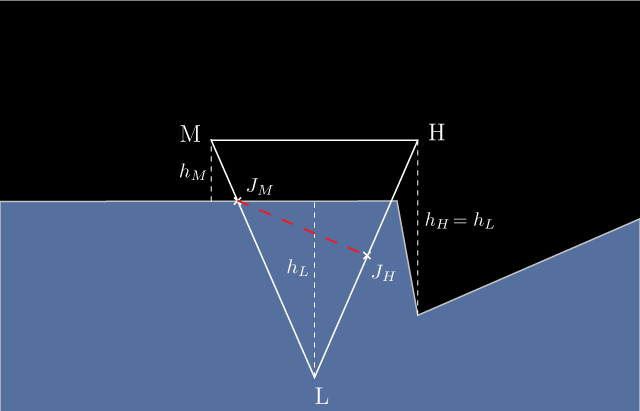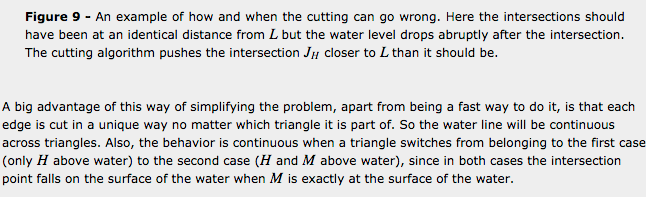## Calculating the hydrostatic forces

Once the cutting algorithm has been run on all triangles of the body's mesh, we have a list of fully submerged triangles. The buoyancy force acting on the body is the sum of all hydrostatic forces acting on each fully submerged triangle. As far as the linear force is concerned, we can sum only the vertical component of the hydrostatic force since we have seen that the other forces cancel each other. The force on a submerged triangle is: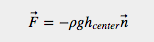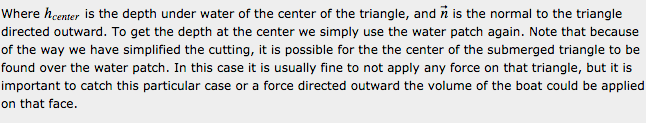Remember that applying the hydrostatic force at the center of the triangle instead of at its real center of application results in a residual torque around the center of the volume displaced. If the number of triangles is low and it is important to not get any residual torque, it is necessary to calculate the point of application of the force on the triangle. Appendix A gives the formula for finding the center of application of the hydrostatic force on two types of triangles which base is horizontal, with the apex either pointing up or pointing down. An arbitrary submerged triangle needs to be further cut in two such triangles, and the two sets of hydrostatic force and centers of application are calculated and summed.

## Piecing it all together

Figure 10 presents an overview of the algorithm.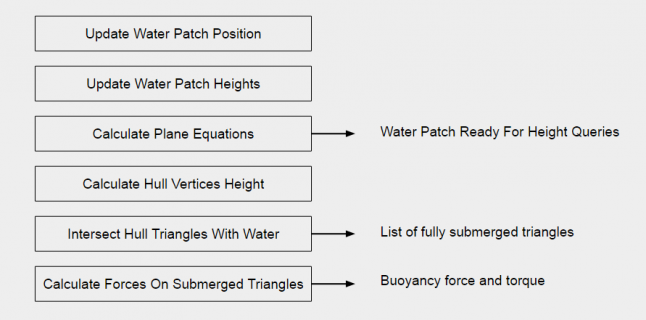Figure 10Outline of the algorithm

So we can summarize the structure of the algorithm as follows (we assume that the x/z plane is the horizontal plane and y is the vertical up axis):

• At each step of the simulation, we update the water patch position to follow the floating body. The water patch does not follow, the vehicle smoothly, as you can see in the animated gif of figure 11. When the boat moves horizontally, it drifts enough from the orginal position that the water patch looses a row (or column) on one side and gains a row (or column) on the opposite side. When this happens, the water patch coordinates (say, its south west corner) changes abruptly from what it was at the preceding step.
• Once we have determined the water patch coordinates, the water height of the water is sampled or calculated at each grid point.
• For each cell we have two triangles formed by the points on the grid elevated in the y direction by the height of the water, as in a traditional height map. We compute the plane equations of the triangles thus formed.
• We then calculate the height above the water of each hull vertex, by determining which triangle it is on top of, and using the plane equation of that triangle
• For each triangle of the mesh, given the height above the water of each of its vertices, we use the cutting algorithm to produce 0, 1 or 2 submerged triangles
• We finally iterate the list of submerged triangles to calculate the water forces (hydrostatic and hydrodynamic)

There are of course lots of optimizations we could do. For instance, we could first project the vertices of the hull vertically to determine exactly which cell triangles are involved, and compute the plane equations only for those triangles.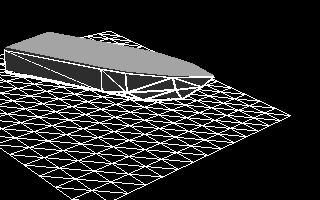Figure 11 - Dynamic response of a boat subjected to hydrostatic forces only, on perfectly flat water. It would get pretty messy in there with a fishing line. It's clear that resistance forces play a big role in reality.

## Conclusion

This article presents an algorithm for intersecting an arbitrary mesh with the surface of water and for calculating the hydrostatic forces acting on the body described by that mesh. If you were to write a program which does only that, you would get a boat oscillating up and down as if it were on a spring. The boat will be pushed out of the water into the air, then fall because of gravity, dive back in and be pushed out again into the air. What is needed to stabilize the system is damping. The hydrodynamic forces which are the subject of the next article are very effective at damping the system, as they are in reality. But it is possible to cheat and just apply heavy speed based damping in all directions of motion, or especially in the vertical dimension, to get a sense of what you get with the hydrostatic forces as calculated with the algorithm we just described.

## Notes

(*) If the pressure were smaller for some reason at a point of a given depth relative to other points at the same depth, water from these neighboring points at the same depth, being subjected to a larger pressure, would flow very quickly to the point with less pressure and reestablish uniform pressure at that depth. The only thing preventing water at a higher pressure at a greater depth from flowing up to a lower pressure at a smaller depth is gravity, which is why there is a gradient of pressure, increasing with depth.

(**) By the way, when taught the theorem in France, it was named the Ostrogradsky theorem. Later I discovered that it was also known as the Gauss theorem. I wonder (half jokingly) if it's because the French are reluctant to attribute too many theorems to the Germans and, given the choice, will gladly go with the Russian equivalent. Americans favor practicality and avoid the whole debate by simply calling it the divergence theorem.

## Appendix A: Hydrostatic Forces on a submerged triangle

To calculate the hydrostatic forces and moments on an arbitrary triangle completely submerged, it is useful to cut the triangle in two smaller triangles, each of which have one edge which is perfectly horizontal. The reason for doing so is that it is much easier to calculate the hydrostatic forces acting on a triangle with a horizontal edge: it can be cut in (practically) rectangular horizontal bands on which surface the pressure is the same everywhere.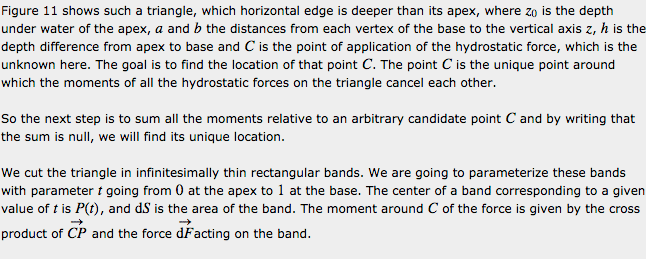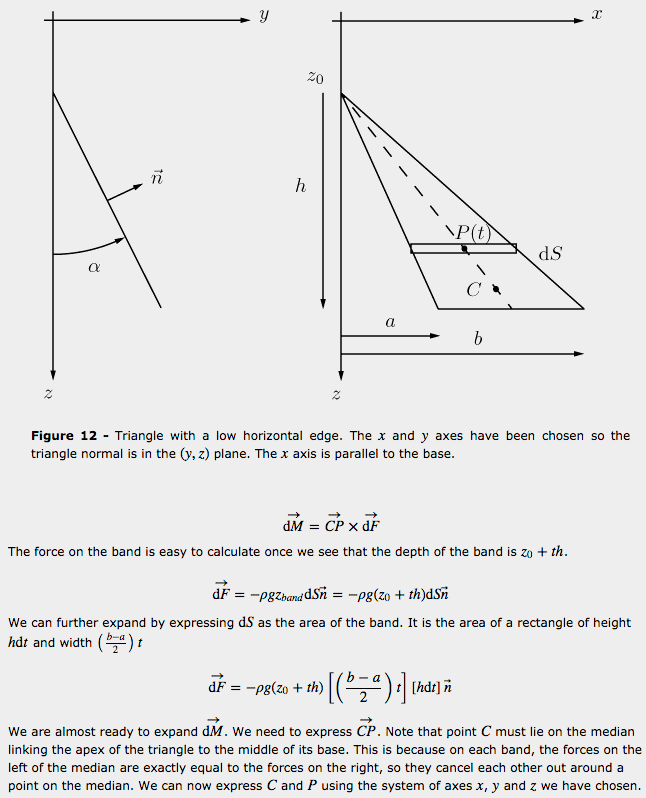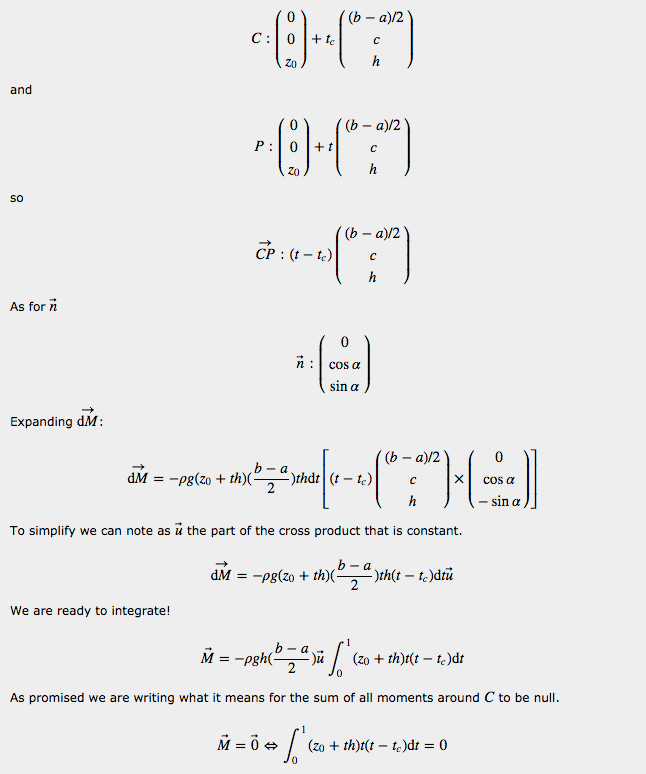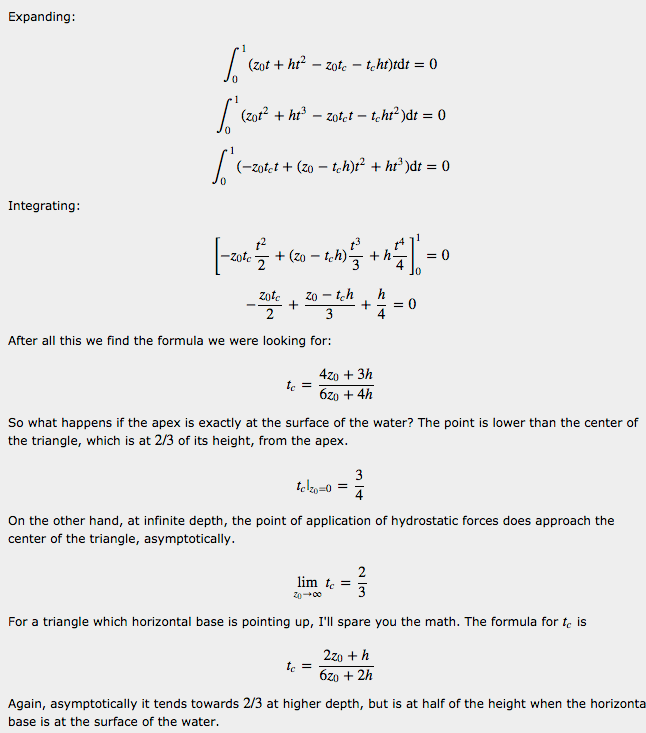## References

1Simship. Edouard Halbert.
2"Buoyancy". Joel Feldman.
3Hydrostatic forces on a plane surface
4Simulating Ocean Water. Jerry Tessendorf.
5Upper Bounds For Packing Of Spheres Of Several Radii. David De Laat, Fernando Mario De Oliveira Filho, Frank Vallentin.

### Latest Jobs

#### Treyarch

Playa Vista, California
6.20.22
Audio Engineer

#### Digital Extremes

6.20.22
Communications Director

6.20.22
Senior Producer

#### Build a Rocket Boy Games

Edinburgh, Scotland
6.20.22
More Jobs